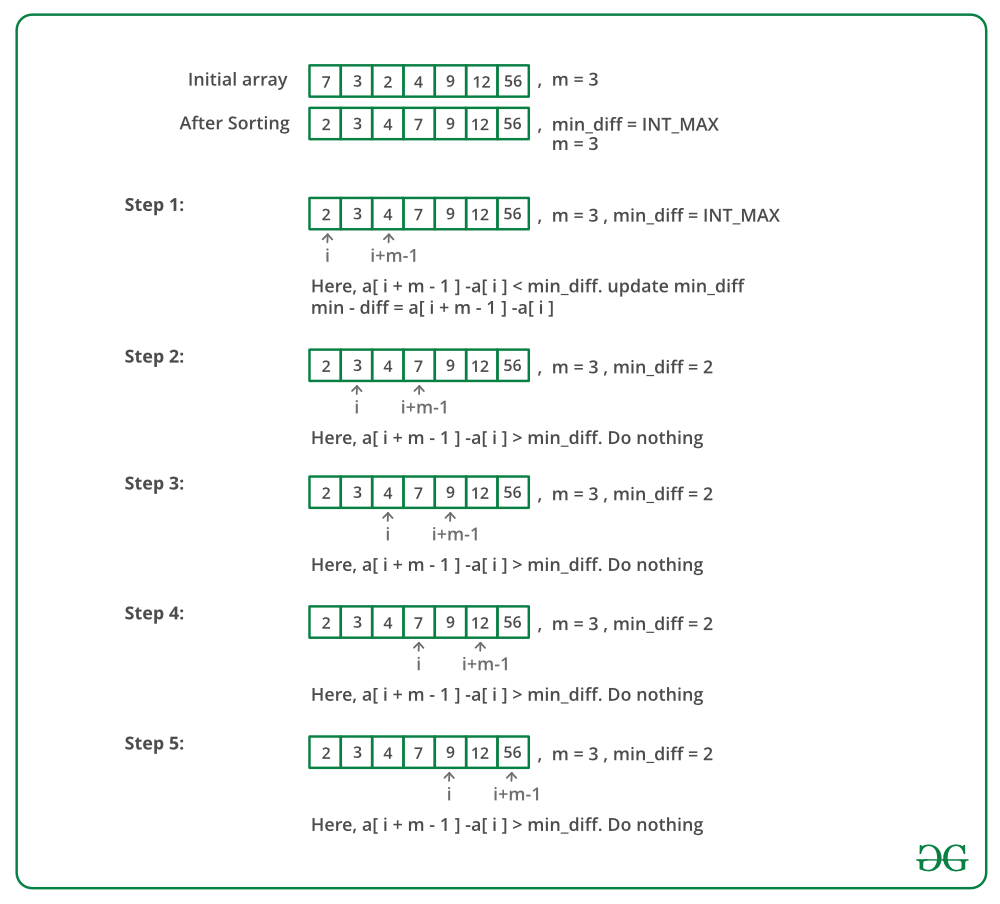# Javascript Program For Chocolate Distribution Problem

Given an array of n integers where each value represents the number of chocolates in a packet. Each packet can have a variable number of chocolates. There are m students, the task is to distribute chocolate packets such that:

1. Each student gets one packet.
2. The difference between the number of chocolates in the packet with maximum chocolates and packet with minimum chocolates given to the students is minimum.

Examples:

Input : arr[] = {7, 3, 2, 4, 9, 12, 56} , m = 3
Output: Minimum Difference is 2
Explanation:
We have seven packets of chocolates and
we need to pick three packets for 3 students
If we pick 2, 3 and 4, we get the minimum
difference between maximum and minimum packet
sizes.

Input : arr[] = {3, 4, 1, 9, 56, 7, 9, 12} , m = 5
Output: Minimum Difference is 6
Explanation:
The set goes like 3,4,7,9,9 and the output
is 9-3 = 6

Input : arr[] = {12, 4, 7, 9, 2, 23, 25, 41,
30, 40, 28, 42, 30, 44, 48,
43, 50} , m = 7
Output: Minimum Difference is 10
Explanation:
We need to pick 7 packets. We pick 40, 41,
42, 44, 48, 43 and 50 to minimize difference
between maximum and minimum.

A simple solution is to generate all subsets of size m of arr[0..n-1]. For every subset, find the difference between the maximum and minimum elements in it. Finally, return the minimum difference.
An efficient solution is based on the observation that to minimize the difference, we must choose consecutive elements from a sorted packet. We first sort the array arr[0..n-1], then find the subarray of size m with the minimum difference between the last and first elements.

Below image is a dry run of the above approach:Below is the implementation of the above approach:

## Javascript

 ``

Output:

`Minimum difference is 10`

Time Complexity: O(n Log n) as we apply sorting before subarray search.

Space complexity: O(1), The algorithm does not require any extra space, so the space complexity is O(1).

Please refer complete article on Chocolate Distribution Problem for more details!

Feeling lost in the world of random DSA topics, wasting time without progress? It's time for a change! Join our DSA course, where we'll guide you on an exciting journey to master DSA efficiently and on schedule.
Ready to dive in? Explore our Free Demo Content and join our DSA course, trusted by over 100,000 geeks!

Previous
Next